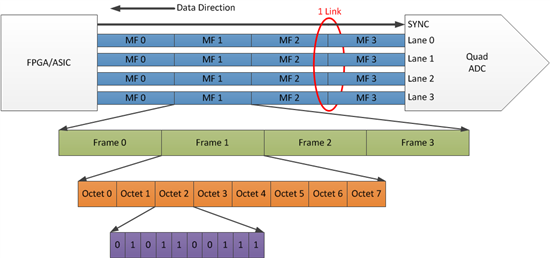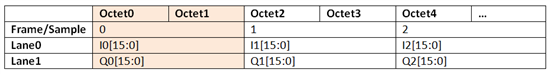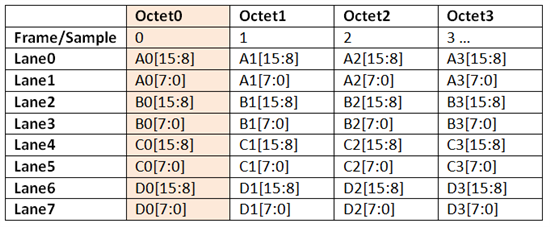# JESD204B: Determining your link configuration

If you’ve been following my JESD204B series, you have a basic understanding of the protocol and signaling required to establish a link between a JESD204B transmitter and receiver. We can now move on to how to configure the JESD204B link with the appropriate parameters for your specific frequency plan.

The main parameters that define a JESD204B link are LMFS and lane rate.

L = number of lanes for the link
M= number of logical converters
F= number of octets per frame
S= number of samples per frame
Lane rate = bits per second per lane

A link is typically comprised of lanes, frames, octets and sample bits. At the highest level, you have 1 link. In Figure 1, the link includes 4 lanes of serial data. Each lane is formed by frames of data, and each frame of data is comprised of a number of octets, with the octets representing the binary sample data.  The question then becomes, how do I specify this link configuration given my system needs?Figure 1. Example of a 16-bit quad ADC with link parameters LMFS=4484. 4 lanes, 4 converters, 8 octets per frame, 2 octets per sample, 4 samples per frame. The 8b octet is shown here as a 10b word just prior to transmission on the SerDes lane.

The idea behind any interface is the transmission of some amount of information from one point to the next – understanding the data throughput requirements (bits per second) and the desired number of lanes will determine the link configuration.

Here’s a real world example to illustrate this process. Let’s assume we’re using the 12-bit, 4GSPS RF-sampling ADC12J4000, which is sampling at 3.1Gsps. The DDC block within the ADC then down converts the ADC samples and decimates the data by 16x. This produces 16-bit complex I and Q data at 193.75Msps. Since the data is 16-bits wide, you’ll need 2 octets per 16-bit sample. The JESD204B standard employs 8b/10b encoding, so each octet will require 10 bits. The total throughput can then be calculated as:

Datarate*Num_Converters*Num_Octets*10bits/Octet=

193.75Msps*2*2*10=7.75Gbps Total throughput

You can then spread this throughput across a number of lanes. For example, if 2 lanes are used, the lane configuration would be LMFS=2221, with each lane at 3.875Gbps.

L=2 lanes
M=2 logical converters (I and Q)
F= 2 octets per frame
S= 1 sample per frame

The resulting ADC link structure would be as follows:Table 1. Frame structure for LMFS=2221, 1 sample across 2 octets

You can apply a similar approach on the digital-to-analog converter (DAC) side as well, since it is just the reverse of the ADC.

For another real world example, let’s take a look at the DAC38J84. We’ll assume the DAC input data rate is 737.28Msps with 4 DAC channels and 16 bits per channel requiring 2 octets/sample.  The amount of data that must be transmitted per sample (throughput) is:

Datarate*Num _Converters*Num_Octets*10bits/octet=

737.28Msps*4*2*10=58.9824Gbps total throughput

You can split this across a number of lanes. If you choose 8 lanes, the lane configuration would be LMFS=8411, with each lane at 7.3728Gbps.

L=8 lanes
M=4 logical converters (Ch A, B, C, D)
F=1 octet per frame
S=1 sample per frame

The resulting DAC link structure for this case would be:Table 2. Frame structure for LMFS=8411, 1 sample per octet, 2 lanes per converter sample

These two examples should provide you with some guidance on how to take your system requirements for data converter sample rate, number of bits and number of channels, and then convert that into JESD204B link configuration parameters.

Be sure to check back next month for my next blog, where I’ll explain JESD204B subclasses and their signaling requirements.

Additional resources:

Parents
• Nice article - Figure 1 is very helpful to clarify the JESD204 data packing scheme.

Comment
• Nice article - Figure 1 is very helpful to clarify the JESD204 data packing scheme.

Children
No Data